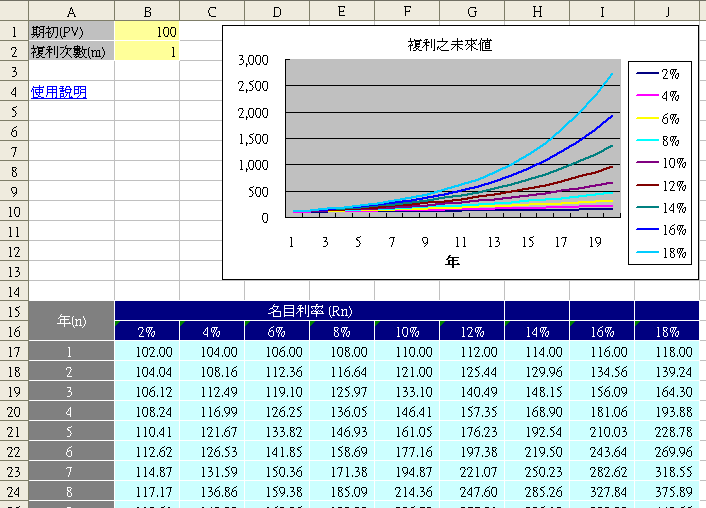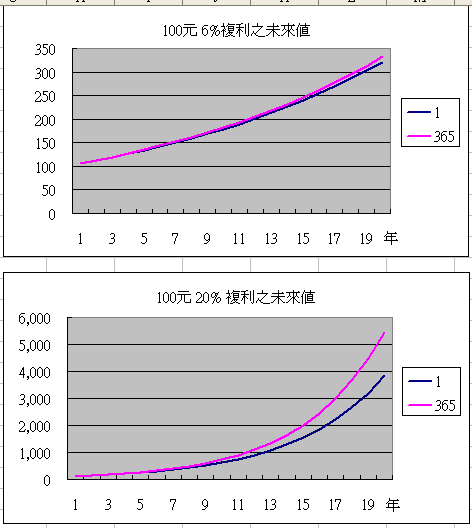﻿ 複利-完全攻略篇

# 單利

= PV + 利息
= PV + PV*Rn*t
= PV*(1+Rn*t)
---------公式(1)

Rn為名目年利率，t 為年數，不足一年以小數或分數表示。例如一季就是(t=1/4)，Rn*t 等於 Rn/4。依此類推一個月(t = 1/12)，一日(t=1/365)，半年(t=1/2)。• 單利
• 複利
• 複利次數之影響
• 實質利率

# 複利## 複利計算公式

FV = PV*(1+rate)n ---------公式(2)2% 18% 1年 102 118 20年 149 2,739

### 複利公式推導

= 期初本金 + 利息
= 期初本金 + 期初本金 *rate
= 期初本金*(1+rate)。

 期數 期初本金 期末終值 (FV) 0 PV 1 PV PV*(1+rate) 2 PV*(1+rate) PV*(1+rate)*(1+rate) = PV*(1+rate)2 3 PV*(1+rate)2 PV*(1+rate)2*(1+rate) = PV*(1+rate)3 .... n PV*(1+rate)n-1 PV*(1+rate)n

FV =1000000*(1+6%)3 =1000000*(1+6%)^3 =1,191,016

## 通用複利公式

FV = PV*(1+Rn/m)m*t ---------公式(3)

• PV：期初金額
• Rn：名目年利率
• m：每年計息次數(複利次數)
• t：年數

Rn/m的意義是每期的利率，例如Rn / 2 就是半年利率；Rn / 4 就是季利率；Rn / 12 就是月利率。m*t就是總計息次數，也就是俗稱的複利次數，例如每月複利一次(m=12)，3年(t=3)總共複利36次 (12*3)。FV = 100*(1+6%/12)36

FV = 100*(1+6%/4)2

# 複利試算

 期初金額(PV) 元 名目利率(Rn) % 複利次數(m) 次 年數(t) 年

## 複利次數對未來值之影響# 實質年利率

 計息 次數 Excel公式 未來值 (FV) 實質年利率 1 =100*(1+6%/1)^1 106.00 6.00% 2 =100*(1+6%/2)^2 106.09 6.09% 4 =100*(1+6%/4)^4 106.14 6.14% 12 =100*(1+6%/12)^12 106.17 6.17% 365 =100*(1+6%/365)^365 106.18 6.18% ∞ =100*exp(6%) 106.18 6.18%

## 連續複利

FV=PV*ｅRn*t

PV是期初值，ｅ是自然指數，Rn 是『名目年利率』，t 是『年』。ｅ在Excel裡用的是EXP函數。

FV = 100*e(6%*2)  以Excel公式表示：

=100*EXP(6%*2)
=112.75

## 實質年利率公式

= 利息 / 本金
= (FV - PV) / PV
= FV/PV - 1 ---------公式(4)

FV可用公式(3)去代入，一年複利終值就是當 t = 1，所以將 FV = PV*(1+Rn/m)m 代入公式(4)

= PV*(1+Rn/m)m/ PV -1
=(1+Rn/m)m- 1
---------公式(5)

Re =>實質利率 (Effect)，Rn=>名目利率(Nominal )，m =>每年複利次數﻿
﻿

■ 本網站內容儘可能精確完整，但不保證無誤。若做為投資依據，風險請自行斟酌 ，本網站不負賠償之責任。
■ 網站所有資料均為版權所有，非經書面允許請勿轉載或使用。